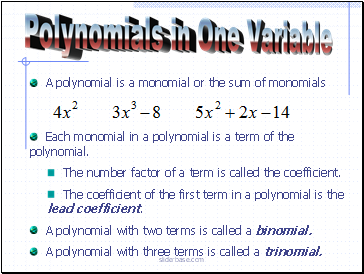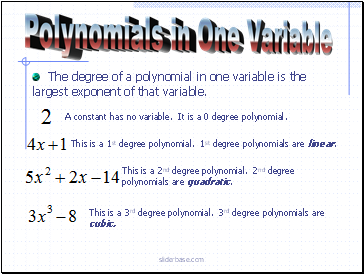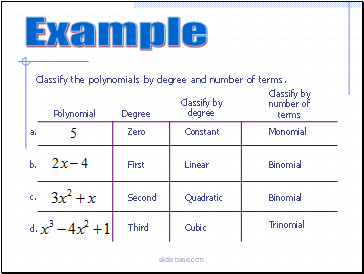# PolynomialsPage 1

#### WATCH ALL SLIDES

Slide 1Polynomials

Slide 2Objectives

Be able to determine the degree of a polynomial.

Be able to classify a polynomial.

Be able to write a polynomial in standard form.

Slide 3## Vocabulary

Monomial: A number, a variable or the product of a number and one or more variables.

Polynomial: A monomial or a sum of monomials.

Binomial: A polynomial with exactly two terms.

Trinomial: A polynomial with exactly three terms.

Coefficient: A numerical factor in a term of an algebraic expression.

Slide 4Vocabulary

Degree of a monomial: The sum of the exponents of all of the variables in the monomial.

Degree of a polynomial in one variable: The largest exponent of that variable.

Standard form: When the terms of a polynomial are arranged from the largest exponent to the smallest exponent in decreasing order.

Slide 5## Degree of a Monomial

What is the degree of the monomial?

The degree of a monomial is the sum of the exponents of the variables in the monomial.

The exponents of each variable are 4 and 2. 4+2 = 6.

The degree of the monomial is 6.

The monomial can be referred to as a sixth degree monomial.

Slide 6## Polynomials in One Variable

A polynomial is a monomial or the sum of monomials

Each monomial in a polynomial is a term of the polynomial.

The number factor of a term is called the coefficient.

The coefficient of the first term in a polynomial is the lead coefficient.

A polynomial with two terms is called a binomial.

A polynomial with three terms is called a trinomial.

Slide 7Polynomials in One Variable

The degree of a polynomial in one variable is the largest exponent of that variable.

A constant has no variable. It is a 0 degree polynomial.

This is a 1st degree polynomial. 1st degree polynomials are linear.

This is a 2nd degree polynomial. 2nd degree polynomials are quadratic.

This is a 3rd degree polynomial. 3rd degree polynomials are cubic.

Slide 8Classify the polynomials by degree and number of terms.

Example

Polynomial

a.

b.

c.

d.

Degree

Classify by degree

Classify by number of terms

Zero

Constant

Monomial

Go to page:
1  2